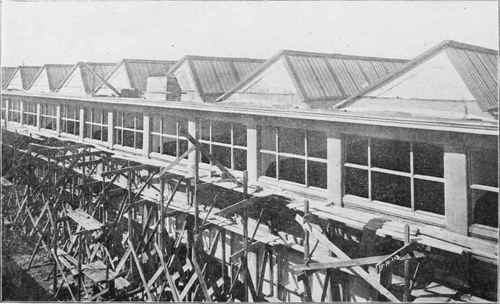Evidently the loads on partsandalso equal 1,920 pounds each; hence the apex loads at joints 1 and 5 equal 960 pounds and at joints 2, 3 and 4, 1,920 pounds.

The wind load must be computed for each slope of the roof separately. The angles whichandmake with the horizontal, scale practically 37 and 15 degrees. According to the table of wind pressures (Art. 19), the pressures for these slopes equal about 35 and 20 pounds per square foot respectively. Since memberis 10 feet long, the wind pressure on the 37-degree slope equals 10 X 12 X 35 = 4,200 pounds.

This force acts perpendicularly to the memberand is to be equally divided between joints 1 and 2 as represented in the figure. Since the memberis 8¼ feet long, the wind pressure on the 15-degree slope equals

8¼ X 12 X 20 = 1,980 or approximately 2,000 pounds.

This pressure acts perpendicularly to memberand is to be equally divided between joints 2 and 3 as represented.

The stress diagram for dead, snow, or wind load for a truss like that represented in Fig. 46, is constructed like those previously explained; but there are a few points of difference in the analysis for wind stress, and these will be explained in what follows.

Example. Let it be required to determine the stresses in the truss of Fig. 46, due to wind loads on the left as represented.

It is necessary to ascertain the reactions due to the wind loads; therefore, find the resultant of the wind pressures, see Art. 37; it equals 6,120 pounds and acts as shown. Now, if both ends of the truss are fastened to the supports, then the reactions are parallel to the resultant wind pressure, and their values can be readily found from moment equations. Let R1 and R2 denote the left and right reactions respectively; then, since the arms of R1 and the resultant wind pressure with respect to the right end equal 27.8 and 19.9 feet respectively,

R1x 27.8 = 6,120 X 19.9 = 121,788 ; hence, R1= 121,788/27.8 = 4,380 pounds approximately.

Since the arms of R2 and the resultant wind pressure with respect to the left support are 27.8 and 7.9 feet respectively,

R2,X 27.8 = 6,120 X 7.9 = 48,348;

 hence, R2 48,348 - 1,740 pounds approximately. 27.8

The next step is to draw the polygon for the loads and reactions ; so we draw lines AB, BC, CD, and DE to represent the loads at joint 1, the two at joint 2, and that at joint 3, respectively ; and then EF to represent the right reaction. (If the reactions have been correctly determined and the drawing accurately done, then FA will represent the left reaction.)

The truss diagram should now be lettered (agreeing with the letters on the polygon just drawn), and then the construction of the stress diagram may be begun. Since this construction presents no points not already explained, it will not be here carried out.

## Example For Practice

Analyze the truss of Fig. 46 for dead, snow, and wind loads as computed in the foregoing, and compute the greatest resultant stress in each member due to combined loads, assuming that the snow and wind do not act at the same time.

## Stress Record

Ans.

 Member. Dead. Snow. Wind Left. Wind Right. Resultant. 12 -3,250 -4,800 -3,450 -2,500 -8,050 23 -2,700 -4,000 - 2,850 -3,100 -6,700 16 +2,600 +3,850 +3,750 +1,150 +6,450 26 0 0 -2,000 +1,250 -2,000 +1,250 36 0 0 + 450 + 450 + 450 46 0 0 +1,250 -2,000 +1,250 -2,000 56 +2,600 +3,850 +1,150 +3,750 +6,450 43 -2,700 -4,000 -3,100 - 2,850 -6,700 54 -3,250 -4,800 -2,500 -3,450 -8,050STEEL FRAME OF BUILDING FOR FIFTH REGIMENT ARMORY, BALTIMORE, MARYLAND.

Span, 200 ft., centers; length of structure, 300 ft.; total weight of steel work, 1,928.000 lbs.

Courtesy of American Bridge Company.PART OF SAWTOOTH ROOF OF ASSEMBLY BUILDING OF THE GEORGE N. PIERCE COMPANY, BUFFALO, N. Y.

Giving a partial view of the 61-foot girders. Kahn System of Reinforced Concrete.

Courtesy of Trussed Concrete Steel Co., Detroit, Mich.